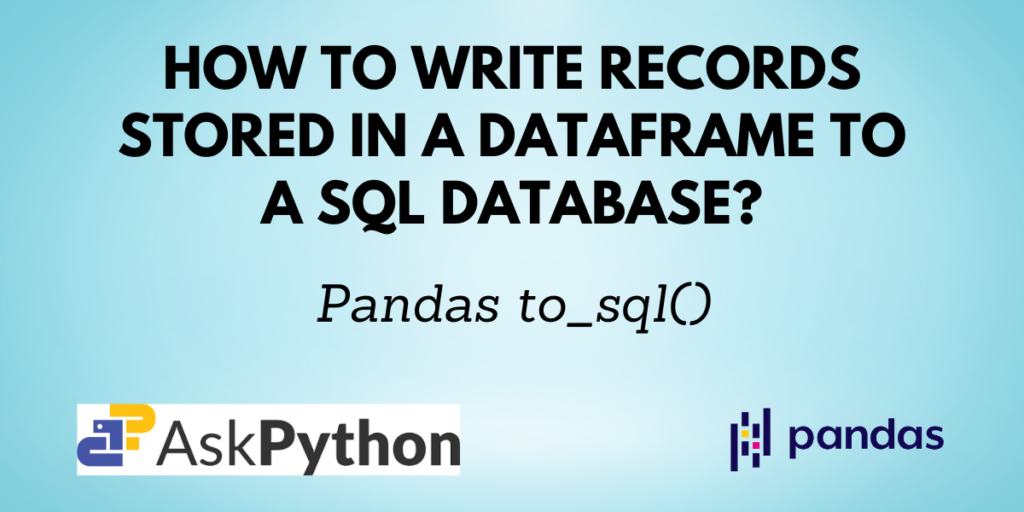# Pandas to_sql(): Write records from a DataFrame to a SQL DatabasePandas is the preferred library for the majority of programmers when working with datasets in Python since it offers a wide range of functions for data cleaning, analysis, and manipulation. You’ll have to use SQL if you incorporate a database into your program. A Pandas DataFrame can be loaded into a SQL database using the `to_sql()` function in Pandas.

You will discover more about the `read_sql()` method for Pandas and how to use it in this article.

## Prerequisites of Pandas to_sql()

Before getting started, you need to have a few things set up on your computer. You need to have Python, Pandas, SQLAlchemy and SQLite and your favorite IDE set up to start coding.

Since SQLAlchemy and SQLite come bundled with the standard Python distribution, you only have to check for Pandas installation. If you do not have it installed by using the command:

```pip install pandas
```

If you are using the Anaconda distribution use the command:

```conda install pandas
```

In this tutorial, we’ll use the file-based database SQLite to set up a connection to a database, add a table, read data from the table, and modify it. This tutorial expects you to know some of the basic SQL commands.

Recommended Read: How to use SQL in Python?

## Syntax of Pandas to_sql()

```DataFrame.to_sql(name, con, schema=None, if_exists='fail', index=True, index_label=None, chunksize=None, dtype=None, method=None)
```

Returns: Number of rows affected by to_sql.

## Implementing the to_sql() method

You have now understood the syntax of the `to_sql()` function. Let me now walk you through how to work with it.

### Step 1: Creating a DataFrame

Let us first create a Pandas DataFrame.

```import pandas as pd

# creating a pandas dataframe
data = {
'Name': ['David', 'Emily', 'Harry'],
'Marks': [84, 63, 92],
'Rank': [2, 3, 1]
}

df = pd.DataFrame(data)

df
```

### Step 2: Establishing a database connection

```from sqlite3 import connect

# create a database and connect to it
conn = connect("student_db.db")
curr = conn.cursor()
```

Here, we have created a database named ‘student_db.db’ in which we will now add a table as shown in the next step.

### Step 3: Add a table to the database

```# create a table
curr.execute('CREATE TABLE IF NOT EXISTS students (Name TEXT, Marks NUMBER, Rank NUMBER)')

# commit the query
conn.commit()
```

In the above code, we have created a table named ‘students’ with the required fields and their data types. Note that, the column names and their data types should match the ones in the Pandas DataFrame.

### Step 4: Use the to_sql() function to write to the database

Now that you have created a DataFarme, established a connection to a database and also added a table to the database, you can use the Pandas `to_sql()` function to write the DataFrame into the database.

```# write the dataframe into the database table
df.to_sql('students', conn, if_exists='replace')
```

Here, the if_exists=’replace’ parameter indicates that replace the table if it already exists in the database.

### Step 5: Fetch all the records from the table

You can verify if the DataFrame was successfully added to the database by fetching all the records from the database table.

```# fetch all the records from the table
curr.execute('''SELECT * FROM students''')

for record in curr.fetchall():
print(record)
```

Output:

```(0, 'David', 84, 2)
(1, 'Emily', 63, 3)
(2, 'Harry', 92, 1)
```

## Conclusion

In this tutorial, you learned about the Pandas `to_sql()` function that enables you to write records from a data frame to a SQL database. You saw the syntax of the function and also a step-by-step example of its implementation.